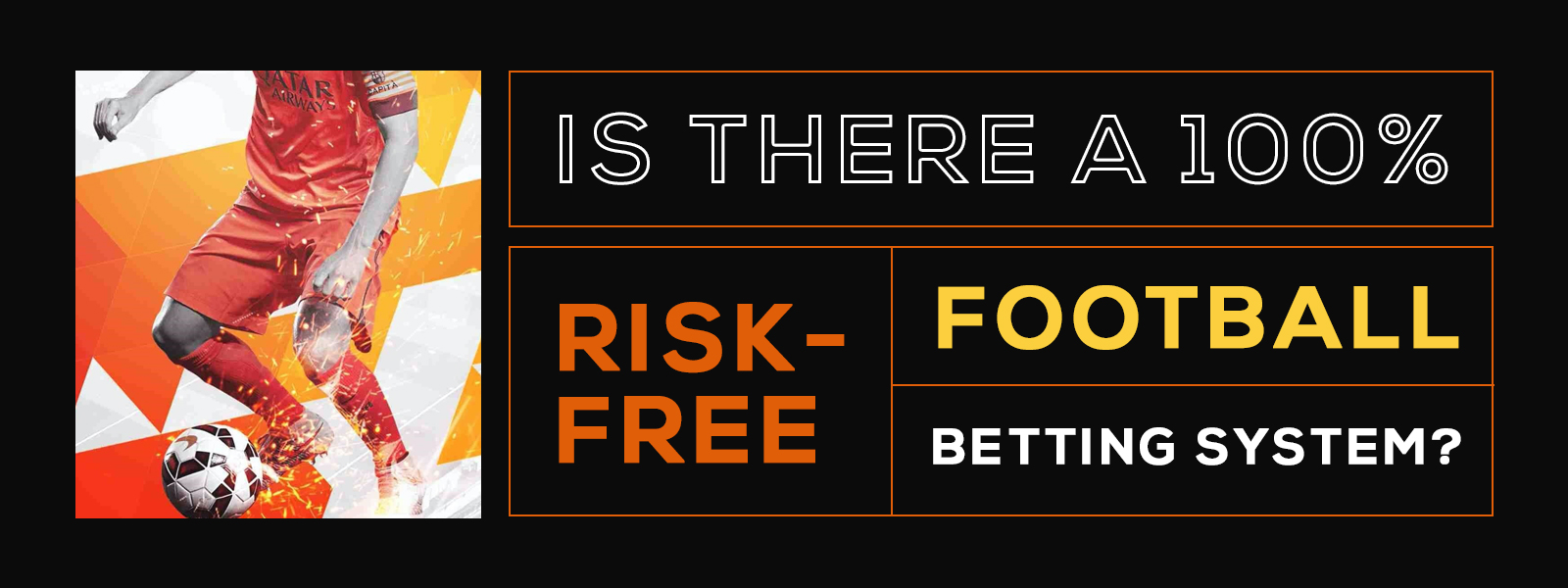Blog
Is There A 100% Risk Free Football Betting System?
Written by, Kristel Gil Wed 09 Dec
Is There A 100% Risk Free Football Betting System?## 100% Risk Free Football Betting System

Risk free football betting is a bettor's dream: winning no matter the match's result. This is not a dream. There is a betting system that will allow you to win for sure when betting on football. This betting system is based on a particular type of bet called surebets or "safe bets". If you can identify them, you can win without risk even before the match starts.

These surebets are not easy to find, but if you do it, you should take advantage of them. You will need to detect a specific condition in the odds. Besides, you must combine your stakes through a particular method to win regardless of the final score. The only way to be able to make surebets is to bet on various online bookmakers. Remember that betting this way; the bookmaker loses, so a unique bookie will not offer you this possibility by itself.

### How To Make Safe Football Bet

To obtain an assured benefit, the sum of the implied probabilities of all possible outcomes must be less than 100%. Remember that the spread represents the bookie profitability and is equal to the surplus of 100% of the sum above. In the example that we are showing we have what is called a negative spread that represents a chance to win for bettors. To carry out this strategy, you must compare odds from several different houses. Or, at least, do it at different moments in time.

Take for example the following odds in an Italian Serie A match:

• Odds:
• Roma's victory: 2.2
• Draw: 4.1
• Fiorentina's win: 4.3

The sum of the implied probabilities yields 93.1%; therefore, we can make a safe bet. Finding the match and the appropriate odds are the first part of the job. The second is to put the right amount of money at each result. Another key to betting with surebets is to conveniently select the amount of money to bet on each possible outcome. To find these amounts, we must establish that the prize obtained with any of the results is the same.

#### Let's See How To Do This

Since the prize is the product between odds and stake, we have:

• (Roma Odds) x (Roma Stake) = (Draw Odds) x (Draw Stake) = (Fiorentina Odds) x (Fiorentina Stake)

We must arbitrarily choose one of the stakes, and from it calculate the other two. If we decide to bet \$ 10 on Roma, we will have the following:

• Draw Stake = (Roma Odds) x (Roma Stake) / Draw Odds
• Fiorentina Stake = (Roma Odds) x (Roma Stake) / Fiorentina Odds

The results would be:

• Draw Stake = 2.2 x 10 / 4.1 = \$ 5.37
• Fiorentina Stake = 2.2 x 10 / 4.3 = \$ 5.12
• Our total bet will be 10 + 5.37 + 5.12 = \$ 20.49. Let's see what happens in each possible outcome:
• If Roma wins, we get a prize of 2.2 x 10 = \$ 22
• If they tie, the prize is 4.1 x 5.37 = \$ 22
• If Fiorentina wins the prize is 4.3 x 5.12 = \$ 22

We have in all three cases prizes of \$ 22, and the total expenses are \$ 20.49. So, whatever happens, we get \$ 1.51. We have illustrated how to find and bet on sure bets. Now, you need to go to your bookies' websites and hunt for them. Then, you will start winning with this 100% risk free betting system.

recent post
categories
tags
Betting Articles